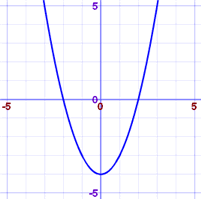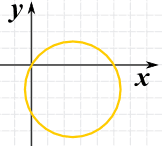# Finding Intercepts From an Equation

X Intercept: where the graph of an equation crosses the x-axis

Y Intercept: where the graph of an equation crosses the y-axisTo find the intercepts:

When you want the x intercepts (x,0):

Set y=0 then solve for x

When you want the y intercepts (0,y):

Set x=0 then solve for y

### Example: Find the intercepts of y = x2 − 4

x intercept: set y=0

0 = x2 − 4

x2 = 4

x = 2 or −2

The points are (2,0) and (−2,0)

y intercept: set x=0

y = 02 − 4

y = −4

The point is (0,-4)

And here is the graph of x2 - 4 to confirm what we found:### Example: Find the intercepts of x2 − 5x + y2 + 3y = 0

x intercept: set y=0

x2 − 5x + 0+ 0 = 0

x(x−5) = 0

x = 0 and 5

The points are (0,0) and (5,0)

y intercept: set x=0

0 − 0 + y2 + 3y = 0

y(y+3) = 0

y = 0 or −3

The points are (0,0) and (0,−3)

So there are a total of 3 points:

(0,0), (5,0) and (0, −3)

And here is the graph ... it's a circle!515, 516, 1152, 1153, 2998, 4039, 4040, 3000, 4041, 4039